# Edexcel A Level Chemistry:复习笔记1.7.4 Yield & Atom Economy

### Yield & Atom Economy

#### Percentage yield

• In a lot of reactions, not all reactants react to form products which can be due to several factors:
• Other reactions take place simultaneously
• The reaction does not go to completion
• Products are lost during separation and purification

• The percentage yield shows how much of a particular product you get from the reactants compared to the maximum theoretical amount that you can get:
•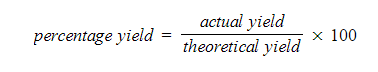• The actual yield is the number of moles or mass of product obtained experimentally
• The theoretical yield is the number of moles or mass obtained by a reacting mass calculation

#### Worked Example

In an experiment to displace copper from copper(II) sulfate, 6.5 g of zinc was added to an excess of copper(II) sulfate solution. The resulting copper was filtered off, washed and dried. The mass of copper obtained was 4.8 g.

Calculate the percentage yield of copper.

Step 1: The balanced symbol equation is:

Zn (s) + CuSO4 (aq) → ZnSO4 (aq) + Cu (s)

Step 2: Calculate the amount of zinc reacted in moles

•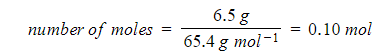Step 3: Calculate the maximum amount of copper that could be formed from the molar ratio:
Since the ratio of  Zn(s) to Cu(s) is 1:1 a maximum of 0.10 moles can be produced
Step 4: Calculate the maximum mass of copper that could be formed (theoretical yield)

mass =   mol  x  M
mass =   0.10 mol x 63.55 g mol-1
mass =   6.4 g (2 sig figs)

Step 5: Calculate the percentage yield of copper

•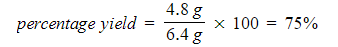#### Atom economy

• The atom economy of a reaction shows how many of the atoms used in the reaction become the desired product
• The rest of the atoms or mass is wasted

• It is found directly from the balanced equation by calculating the Mr of the desired product
•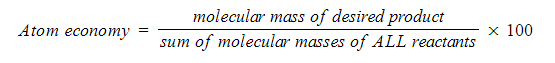• In addition reactions, the atom economy will always be 100% because all of the atoms are used to make the desired product
• Whenever there is only one product, the atom economy will always be 100%
• For example, in the reaction between ethene and bromine:

CH2=CH2 + Br2 → CH2BrCH2Br

• The atom economy could also be calculated using mass, instead or Mr
• In this case, you would divide the mass of the desired product formed by the total mass of all reactants, and then multiply by 100
• Questions about atom economy often ask in qualitative or quantitative terms

#### Worked Example

Qualitative atom economy
Ethanol can be produced by various reactions, such as:

Hydration of ethene:       C2H4 + H2O → C2H5OH

Substitution of bromoethane:    C2H5Br + NaOH → C2H5OH + NaBr

Explain which reaction has a higher atom economy.

Hydration of ethene has a higher atom economy (of 100%) because all of the reactants are converted into products, whereas the substitution of bromoethane produces NaBr as a waste product

#### Worked Example

Quantitative atom economy

The blast furnace uses carbon monoxide to reduce iron(III) oxide to iron.

Fe2O3 + 3CO → 2Fe + 3CO2

Calculate the atom economy for this reaction, assuming that iron is the desired product.

(Ar / Mr data: Fe2O3 = 159.6, CO = 28.0, Fe = 55.8, CO2 = 44.0)

Step 1: Write the equation:

•Step 2: Substitute values and evaluate:

•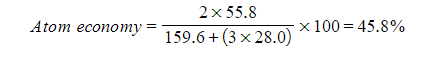#### Exam Tip

Careful: Sometimes a question may ask you to show your working when calculating atom economy. In this case, even if it is an addition reaction and it is obvious that the atom economy is 100%, you will still need to show your working.